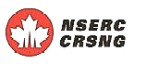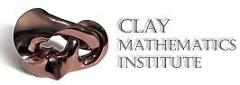## SCIENTIFIC PROGRAMS AND ACTIVITIESJune  7, 2023THE FIELDS INSTITUTE FOR RESEARCH IN MATHEMATICAL SCIENCES
 FOCUSED RESEARCH WORKSHOP ON EXTERIOR DIFFERENTIAL SYSTEMS AND LIE THEORY December 9-13, 2013 at the Fields Institute , 222 College St. Toronto Organizing Committee Rui Loja Fernandes, (UIUC), Niky Kamran (McGill), Peter J. Olver (Minnesota)Anthony D. Blaom (AUT)
Cartan connections on Lie algebroids

A Lie groupoid equipped with a multiplicatively closed, horizontal connection may be viewed as a symmetry deformed by curvature'. These connections --- which we call {\em Cartan} connections --- are related to the connections on principal bundles of the same name. However, unlike their classical namesakes, no homogeneous model is fixed beforehand and intransitivity is allowed. Most significantly, Cartan connections can be infinitesimalized to obtain certain linear connections on the corresponding Lie algebroid. This introductory talk discusses these latter connections, explaining the sense in which they are infinitesimal symmetries deformed by curvature. We sketch the construction of the canonical Cartan connection for Riemannian geometry, and mention some applications to uniformization problems.

Robert Bryant (Duke University)

Henrique Bursztyn (IMPA)
Multiplicative geometric structures on Lie groupoids

In their many applications, Lie groupoids often come equipped with additional geometric data (e.g. symplectic forms, Poisson structures, complex structures, distributions etc) which are compatible with the groupoid structure, known as "multiplicative". This talk will discuss a general result describing multiplicative geometric structures at the infinitesimal level (i.e., in terms of Lie algebroids),
with focus on examples.

Marius Crainic (Utrecht University)

Marco Gualtieri (Toronto)

Irina Kogan (North Carolina State University and IMA)
Invariant Variational Calculus

Many interesting variational problems arising in geometry and physics admit a group of symmetries. As first recognized by S. Lie, these problems can be rewritten in terms of group-invariant objects: differential invariants, invariant differential forms, and invariant differential operators. It is desirable from both computational and theoretical points of view to perform variational calculus in terms of invariant objects. In this talk, we will discuss invariant variational bicomplex, invariant Euler-Lagrange operators and invariant Noether correspondence.

Boris Kruglikov (Tromso)

Kirill Mackenzie (Sheffield)
Multiple differentiation processes in differential geometry

Taking the Lie algebroid of a Lie groupoid encompasses many of the basic differentation processes in differential geometry.
In this talk we give an overview of the second--order version of this process, in which double Lie groupoids give rise, by two differentiation processes, to double Lie algebroids. These encompass iterated tangent bundles and their duals, and the classical canonical diffeomorphisms between them extend to double Lie algebroids and their duals.
The cotangent bundle of a Poisson Lie group yields by differentiation the Drinfel'd double of its tangent Lie bialgebra, and may be integrated to a (symplectic) double Lie groupoid. Thus Poisson Lie groups are the intermediate stage in the process of taking the double Lie algebroids of symplectic double Lie groupoids. We describe this, and its extension to Poisson groupoids and Lie bialgebroids.
In conclusion we outline the supergeometric formulation of double Lie algebroids due to Th. Voronov, which extends naturally to $n$-fold Lie algebroids.
References:
1) K. C. H. Mackenzie.Ehresmann doubles and Drinfel'd doubles for Lie algebroids and Lie bialgebroids.
J. Reine Angew. Math., 658:193--245, 2011.
2) K.C.H. Mackenzie. Double Lie algebroids and second-order geometry. II. Adv. Math., 154(1):46--75, 2000.
3) Th.Th. Voronov. Q-Manifolds and Mackenzie Theory. Comm. Math. Phys, 315(2):279--310, 2012.

Eckhard Meinrenken (University of Toronto)
On the Van Est homomorphism for Lie groupoids

Let G be a Lie groupoid, and A the corresponding Lie algebroid. In their 1991 article, Weinstein-Xu described a Van Est map from the complex of smooth groupoid cochains on G to the Chevalley-Eilenberg Lie algebroid complex of A. It is an algebra morphism on the normalized cochains. In this talk, we will give an explanation of this Van Est map in terms of the fundamental lemma of homological pertubation theory. (Joint work with David Li-Bland.)

Eva Miranda (Universitat Politècnica de Catalunya)
Symmetries of b-manifolds and their generalizations

The aim of this talk is to show some examples of simple Poisson manifolds which have some common features with symplectic manifolds (including the study of group actions). I will start presenting a Delzant theorem for toric b-symplectic manifolds (joint work with Victor Guillemin, Ana Rita Pires and Geoffrey Scott).
Time permitting, I will report on an ongoing project with Geoffrey Scott on generalizing the notion of b-symplectic manifold. This notion includes other Poisson manifolds which share good properties with b-symplectic manifolds and seem to have less topological constraints.

Ercument Ortacgil (Bogazici University)
Higher order characteristic classes

Using the theory of prehomogeneous geometries that we defined earlier, we outline the construction of the higher order Pontryagin classes as obstructions to local homogeneity. The problem whether these invariants can be nontrivial remains to be studied.

Juha Pohjanpelto (Oregon State and Aalto Universities)
Group Actions and Cohomology in the Calculus of Variations

I will discuss various new techniques based in part on the moving frames method and Lie algebra cohomology for analyzing the cohomology of a variational bicomplex and the associated edge, or Euler-Lagrange, complex invariant under a continuous pseudo-group action. In particular, these methods in many situations reduce the computation of the local cohomology of the two complexes to an algebraic problem.

I will illustrate the new techniques with examples pertaining to $G$-structures and integrable systems.

Colleen Robles, IAS/TAMU
Schubert varieties as variations of Hodge structure.

Variations of Hodge structure (VHS) are constrained by a system of differential equations known as the infinitesimal period relation (IPR), or Griffiths transversality. The IPR is a homogeneous system defined on a flag manifold X = G/P. I will characterize the Schubert varieties that arise as variations of Hodge structure (VHS). I will also discuss the central role that these Schubert VHS play in our study of arbitrary VHS: infinitesimally their orbits under the isotropy action span' the space of all VHS. This yields a complete description of the infinitesimal VHS. As a corollary we obtain sharp bounds on the maximal dimension of a VHS, answering a longstanding question.

Maria Amelia Salazar,CRM-Barcelona

Abraham Smith (Fordham)

Ivan Struchiner (University of São Paulo)

Francis Valiquette (Dalhousie University)

Ori Yudilevich (Utrecht University)

Nguyen Tien Zung, University of Toulouse
Deformation coholomogy of singular foliations

The aim of this talk is to introduce the deformation cohomology for singular foliations, to show how it's related to stability and deformation problems of foliations, and how to compute it in various cases.

Top